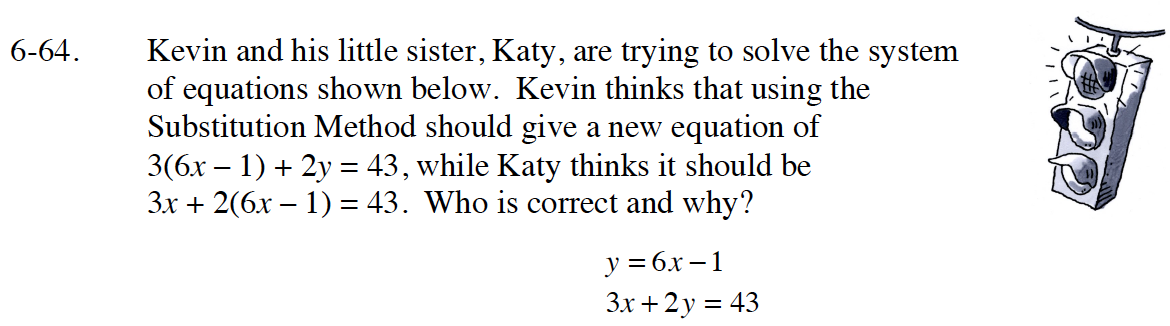Home > GB8I > Chapter cc46 > Lesson cc46.2.2 > Problem6-64

6-64.
1.Kevin and his little sister, Katy, are trying to solve the system of equations shown below. Kevin thinks that using the Substitution Method should give a new equation of 3(6x – 1) + 2y = 43, while Katy thinks it should be 3x + 2(6x − 1) = 43. Who is correct and why? Homework Help ✎

y = 6x − 1
3x + 2y = 43In order to solve the system of equations 6x - 1 must be substituted in for y.

3x + 2( 6x - 1 ) = 43

Katy is right.